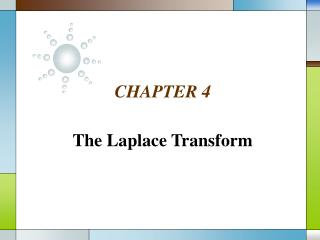DownloadDownload PresentationCHAPTER 4

# CHAPTER 4

Download Presentation## CHAPTER 4

- - - - - - - - - - - - - - - - - - - - - - - - - - - E N D - - - - - - - - - - - - - - - - - - - - - - - - - - -
##### Presentation Transcript

1. CHAPTER 4 The Laplace Transform

2. Contents • 4.1 Definition of the Laplace Transform • 4.2 The Inverse Transform and Transforms of Derivatives • 4.3 Translation Theorems • 4.4 Additional Operational Properties • 4.5 The Dirac Delta Function

3. 4.1 Definition of Laplace Transform DEFINITION 4.1 If f(t)is defined for t  0,then (2)is said to be the Laplace Transform of f. Laplace Transform • Basic DefinitionIf f(t)is defined for t  0,then the improper integral(1)

4. Example 1 Evaluate L{1} Solution: Here we keep that the bounds of integral are 0 and  in mind.From the definition , s>0Since e-st 0as t ,for s > 0.

5. Example 2 Evaluate L{t} Solution , s>0

6. Example 3 Evaluate L{e-3t} Solution

7. Example 4 Evaluate L{sin2t} Solution

8. Example 4 (2) Laplace transform of sin 2t ↓

9. L.T. is Linear • We can easily verify that(3)

10. THEOREM 4.1 (a) (b) (c) (d) (e) (f) (g) Transform of Some Basic Functions

11. DEFINITION 4.2 A function f(t)is said to be of exponential order, if there exists constants c>0, M > 0, and T > 0,such That |f(t)|  Mect for all t > T. See Fig 4.2, 4.3. Exponential Order

12. Fig 4.2

13. Examples See Fig 4.3

14. Fig 4.4 • A function such as is not of exponential order, see Fig 4.4

15. THEOREM 4.2 If f(t) is piecewise continuous on [0, ) and of exponential order, then L{f(t)}exists for s > c. Sufficient Conditions for Existence

16. Fig 4.1

17. Example 5 Find L{f(t)}for Solution

18. 4.2 If F(s)=L(f(t)), then f(t) is the inverse Laplace transform of F(s) and f(t)=L(F(s)) THEOREM 4.3 (a) (b) (c) (d) (e) (f) (g) Some Inverse Transform

19. Example 1 Find the inverse transform of (a) (b) Solution(a)(b)

20. L -1 is also linear • We can easily verify that(1)

21. Example 2 Find Solution(2)

22. Example 3: Partial Fraction Find SolutionUsing partial fractionsThen (3)If we set s = 1, 2, −4,then

23. Example 3 (2) (4)Thus (5)

24. Uniqueness of L -1 • Suppose that the functions f(t) and g(t) satisfy the hypotheses of Theorem 4.2, so that their Laplace transform F(s) and G(s) both exist. If F(s)=G(s) for all s>c (for some c), then f(t)=g(t) whenever on [0, + ) both f and g are continuous.

25. Transform of Derivatives , s>0 (6) , s>0 (7) (8)

26. THEOREM 4.4 If are continuous on [0, ) and are of Exponential order and if f(n)(t) is piecewise-continuous On [0, ), thenwhere Transform of a Derivative

27. Solving Linear ODEs • Then(9)(10)

28. We have(11)where

29. Example 4: Solving IVP Solve Solution(12)(13)

30. Example 4 (2) We can find A = 8, B = −2, C = 6Thus

31. Example 5 Solve Solution(14)Thus

32. 4.3 Translation Theorems THEOREM 4.5 Proof If f is piecewise continuous on [0, ) and of exponential order, then limsL{f} = 0. Behavior of F(s) as s → 

33. THEOREM 4.6 ProofL{eatf(t)} = e-steatf(t)dt = e-(s-a)tf(t)dt = F(s – a): replacing all s in F(s) by s-a If L{f} = F(s) and a is any real number, then L{eatf(t)} = F(s – a), See Fig 4.10. Translation on the s-axis

34. Fig 4.10

35. Example 1 Find the L.T. of(a) (b) Solution(a)(b)

36. Inverse Form of Theorem 4.6 • (1)where

37. Parttial Fraction • To perform the inverse transform of R(s)=P(s)/Q(s): • Rule 1: Linear Factor Partial Fractions • Rule 2: Quadratic Factor Partial Fractions

38. Example 2 Find the inverse L.T. of(a) (b) Solution(a) we have A = 2, B = 11 (2)

39. Example 2 (2) And(3)From (3), we have(4)

40. Example 2 (3) (b) (5) (6) (7)

41. Example 3 Solve Solution

42. Example 3 (2) • (8)

43. Example 4 Solve Solution

44. Example 4 (2)

45. DEFINITION 4.3 The Unit Step Function U(t – a) defined for is Unit Step Function See Fig 4.11.

46. Fig 4.11

47. Fig 4.12 Fig 4.13 • Fig 4.12 shows the graph of (2t – 3)U(t – 1).Considering Fig 4.13, it is the same as f(t) = 2 –3U(t – 2) +U(t – 3),

48. Also a function of the type(9)is the same as(10)Similarly, a function of the type(11)can be written as (12)

49. Example 5 Express in terms of U(t). See Fig 4.14. SolutionFrom (9) and (10), with a = 5, g(t) =20t, h(t) = 0 f(t) =20t – 20tU(t – 5)